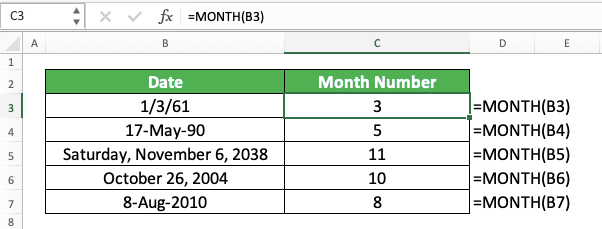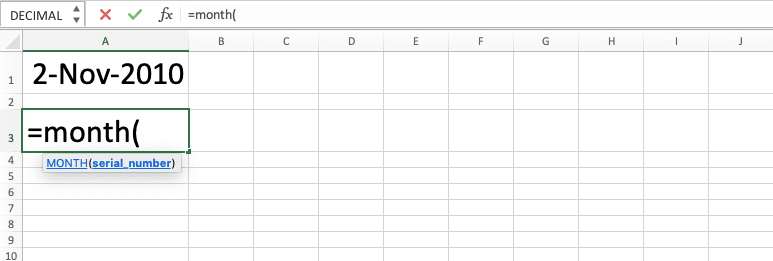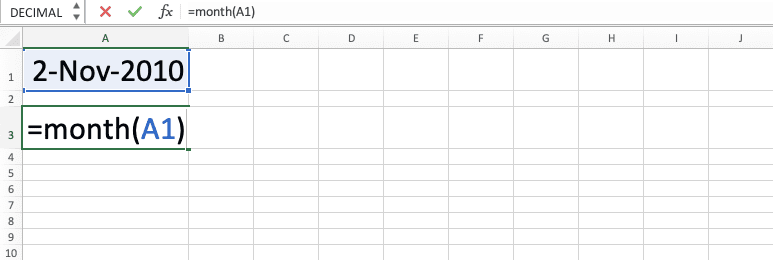How to Use the MONTH Formula in Excel: Functions, Examples, and Writing Steps - Compute Expert

# How to Use the MONTH Formula in Excel: Functions, Examples, and Writing Steps

Home >> Excel Tutorials from Compute Expert >> Excel Formulas List >> How to Use the MONTH Formula in Excel: Functions, Examples, and Writing Steps

In this tutorial, you will learn how to use the MONTH formula in excel completely.

When working with dates in excel, we sometimes need to get the month number of the dates we have. We might need that number for our further data processing or to get the results we want from our excel work.

If we know the way to use the MONTH formula in excel, then we can get that month number easily. Want to know more about MONTH and master the way to use this function properly in excel? Read this tutorial until its last part!

Disclaimer: This post may contain affiliate links from which we earn commission from qualifying purchases/actions at no additional cost for you. Learn more

## What is the MONTH Formula in Excel?

The MONTH formula in excel is a formula that helps you to get the month number of a date.

## MONTH Function in Excel

You can use MONTH to extract a date’s month number in excel.

## MONTH Result

The MONTH result is a number that represents the month number of a date you input into it.

## Excel Version from Which We Can Start Using MONTH

We can start using MONTH in excel since excel 2003.

## The Way to Write It and Its Input

Here is the general writing form of MONTH in excel.

= MONTH ( date )

When using MONTH, you just have to input the date from which you want to get your month number.

## Example of Its Usage and Result

Here is a usage and result example of MONTH in excel.As you can see in the example, the way to use MONTH to get our month number is quite easy. Just input the date we want to get the month number from and press enter. By doing that, we will immediately get the result we want.

## Writing Steps

Need guidance when you write your MONTH formula in excel? See these MONTH writing steps below!

1. Type an equal sign ( = ) in the cell where you want to put the month number of your date2. Type MONTH (can be with large and small letters) and an open bracket sign after =3. Input the date from which you want to get your month number4. Type a close bracket sign5. Press Enter
6. Done!## Get Current Month in Excel: MONTH TODAY

Need to get the current month number in excel? You can get it easily if you combine the excel MONTH and TODAY formulas!

Here is the general writing form of the two formulas combination for the purpose.

= MONTH ( TODAY () )

Simple, isn’t it? Just write the TODAY formula inside your MONTH formula. As TODAY gives you today’s date, MONTH will automatically give you the current month from the date!

Here is an implementation example of the MONTH and TODAY formulas combination in excel.By combining MONTH and TODAY, we will immediately get the current month number in excel!

Be aware, though, that the current month number will keep updating itself to the latest today’s date. This is the nature of TODAY in excel, which keeps updating its result each time you open or edit its worksheet.

If you want a static current month instead, just paste values your MONTH TODAY formula writing.

To do that, press Ctrl + C when you highlight the cell that contains the MONTH TODAY formula writing. Then, right-click on the same cell (or go to the Home tab and click the Paste dropdown button there), highlight Paste Special, and choose Values.Then, press the Escape button to remove the Copy mode from your cell. By doing that, you have got a static current month number in your cell (by removing the MONTH TODAY formula writing and getting only its result though the paste value feature utilization)!

Need the current month name instead of its number? Follow the next part of this tutorial to convert the current month number to its name!

## Get the Month Name from a Date in Excel: CHOOSE MONTH

Want to get the month name of a date instead of its month number? Well, you should still get its month number first using MONTH. After that, you can convert that number to the month name by using the CHOOSE formula.

Here is the general writing form of the CHOOSE and MONTH formulas combination for the month name retrieval purpose.

= CHOOSE ( MONTH ( date ) , “January” , “February” , “March” , “April” , “May” , “June” , “July” , “August” , “September” , “October” , “November” , “December” )

CHOOSE is a formula which first input (a number) determines the result it gives based on the result options it has.

In this formula writing, we use the date month number from MONTH as the CHOOSE first input. Then, we input the result options that consist of all month names in a year, from January to December.

By doing that, the date month number from MONTH will determine the month name we get from CHOOSE. Thus, we will get the correct month name of our date!

Here is the CHOOSE and MONTH formulas combination implementation example in excel.Need the month name of your date in excel? Combine CHOOSE and MONTH formulas to get your month name fast and easily!

## Exercise

After you have understood how to use MONTH in excel completely, let’s deepen your understanding further by doing the exercise below!

### Questions

Answer each of these questions on the appropriate gray-colored cells!
1. What is the content of each “Production Month” column cell?
2. What is the content of each “Selling Month” column cell?
3. How many are the monthly sales quantities of the company from January to December? Use the SUMIF formula to help you in answering this question by also utilizing your answer to the previous question!

You can also get a month number result if you input a number from 1 to 2958465 to MONTH. That is because a date is equivalent to a specific number in excel.

Related tutorials you should learn from:

Get updated excel info from Compute Expert by registering your email. It's free!